# CBSEtips.in

## Wednesday 24 February 2021

### CBSE Class 8 Maths - MCQ and Online Tests - Unit 11 - Mensuration

#### CBSE Class 8 Maths – MCQ and Online Tests –  Unit 11 – Mensuration

Every year CBSE students attend Annual Assessment exams for 6,7,8,9,11th standards. These exams are very competitive to all the students. So our website provides online tests for all the 6,7,8,9,11th standards’ subjects. These tests are also very effective and useful for those who preparing for any competitive exams like Olympiad etc. It can boost their preparation level and confidence level by attempting these chapter wise online tests.

These online tests are based on latest CBSE syllabus. While attempting these, our students can identify their weak lessons and continuously practice those lessons for attaining high marks. It also helps to revise the NCERT textbooks thoroughly

#### CBSE Class 8 Maths – MCQ and Online Tests – Unit 11 – Mensuration

Question 1.
The perimeter of the figure is(a) 4 cm
(b) 6 cm
(c) 8 cm
(d) 12 cm.

Hint:
Perimeter = 4 Ũ 2 = 8 cm.

Question 2.
If a cuboidal box has height, length and width as 20 cm, 15 cm and 10 cm respectively. Then its total surface area is:
(a) 1100 cm2
(b) 1200 cm2
(c) 1300 cm2
(d) 1400 cm2

Hint:
Total surface area = 2 (20 Ũ 15 + 20 Ũ 10 + 10 Ũ 15)
TSA = 2 ( 300 + 200 + 150) = 1300 cm2

Question 3.
1 L =
ia) 10 cmģ
(b) 100 cmģ
(c) 1000 cmģ
(d) 10000 cmģ.

Question 4.
1 mģ =
(a) 1 L
(b) 10 L
(c) 100 L
(d) 1000 L.

Question 5.
The area of the figure is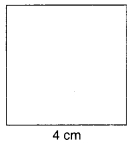(a) 16 cmē
(b) 8 cmē
(c) 4 cmē
(d) 12 cmē

Hint:
Area = 4 Ũ 4 = 16 cmē.

Question 6.
The area of the figure is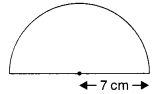(a) 77 cmē
(b) 154 cmē
(c) 38.5 cmē
(d) none of these

Hint:
Area = $$\frac{1}{2}$$ Ũ $$\frac{22}{7}$$ Ũ 7 Ũ 7 = 77 cmē.

Question 7.
The diagram has the shape of a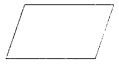(a) rectangle
(b) square
(c) parallelogram
(d) circle.

Question 8.
The diagram has the shape of a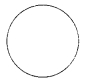(a) circle
(b) square
(c) rectangle
(d) parallelogram.

Question 9.
The diagram has the shape of a(a) circle
(b) parallelogram
(c) rectangle
(d) trapezium.

Question 10.
The area of a parallelogram of base b and altitude h is
(a) $$\frac{1}{2}$$bh
(b) bh
(c) $$\frac{1}{3}$$bh
(d) $$\frac{1}{4}$$bh.

Question 11.
The area of a circle of radius r is
(a) $$\frac{1}{2}$$prē
(b) rē
(c) prē.
(d) $$\frac{1}{4}$$prē

Question 12.
1 cmģ =
(a) 1000 mmģ
(b) 100 mmģ
(c) 10 mmģ
(d) $$\frac{1}{1000}$$mmģ

Question 13.
1 mģ =
(a ) 1000000 cmģ
(b) 100 cmģ
(c) 10 cmģ
(d) $$\frac{1}{1000}$$ cmģ

Question 14.
1 mmģ =
(a) 0.001 cmģ
(b) 0.01 cmģ
(c) 0.1 cmģ
(d) 1000 cmģ

Question 15.
1 cmģ =
(a) 0.000001 mģ
(b) 0.01 mģ
(c) 0.1 mģ
(d) 1000 mģ

Question 16.
The surface area of a cuboid of length l, breadth b and height h is
(a ) lbh
(b) lb + bh + hl
(c) 2 (lb + bh + hl)
(d) 2(l + b)h.

Answer: (c) 2 (lb + bh + hl)

Question 17.
The surface area of a cube of edge a is
(a) 4aē
(b) 6aē
(c) 3aē
(d) aē.

Question 18.
The total surface area of a cylinder of base radius r and height h is
(a) 2pr (r + h)
(b) pr (r + h)
(c) 2prh
(d) 2prē.

Answer: (a) 2pr (r + h)

Question 19.
The volume of a cuboid of length l, breadth b and height h is
(a) lbh
(b) lb + bh + hl
(c) 2 (lb + bh + hl)
(d) 2 (l + b) h.

Question 20.
The volume of a cube of edge a is
(a) aē
(b) aģ
(c) a4
(d) 6aē

Question 21.
The volume of a cylinder of base radius r and height h is
(a) 2prh
(b) prēh
(c) 2pr (r + h)
(d) $$\frac{1}{3}$$ prēh.

Question 22.
The perimeter of the figure is(a) 7 cm
(b) 14 cm
(c) 12 cm
(d) 24 cm.

Hint:
Perimeter = 2 (4 + 3) = 14 cm.

Question 23.
The diagram has the shape of a(a) square
(b) rectangle
(c) triangle
(d) trapezium.

Question 24.
The diagram has the shape of a(a) rectangle
(b) square
(c) circle
(d) parallelogram.

Question 25.
The diagram has the shape of a(a) circle
(b) rectangle
(c) square
(d) triangle.

Question 26.
The area of the figure is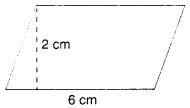(a) 8 cmē
(b) 6 cmē
(c) 12 cmē
(d) 16 cmē

Hint:
Area = 6ē = 12 cmē.

Question 27.
The perimeter of the figure is(a) 5 cm
(b) 10 cm
(c) 4 cm
(d) 8 cm.

Hint:
Perimeter = 2 (4 + 1) = 10 cm.

Question 28.
The area of the figure is(a) 6 cmē
(b) 12 cmē
(c) 5 cmē
(d) 10 cmē

Hint:
Area = 3 Ũ 2 = 6 cmē.

Question 29.
The perimeter of the figure is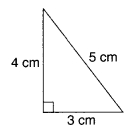(a) 12 cm
(b) 24 cm
(c) 6 cm
(d) 60 cm.

Hint:
Perimeter = 4 + 3 + 5 = 12 cm.

Question 30.
The area of the figure is(a) 9 cmē
(b) 18 cmē
(c) 12 cmē
(d) 15 cmē

Hint:
Area = $$\frac{6Ũ3}{2}$$ 9 cmē.

Question 31.
The height of a cylinder whose radius is 7 cm and the total surface area is 968 cm2 is:
(a) 15 cm
(b) 17 cm
(c) 19 cm
(d) 21 cm

Hint:
Total surface area = 2pr (h + r)
968 = 2 x 22/7 x 7 (7+h)
h = 15 cm

Question 32.
The height of a cuboid whose volume is 275 cm3 and base area is 25 cm2 is:
(a) 10 cm
(b) 11 cm
(c) 12 cm
(d) 13 cm

Hint:
Volume of a cuboid = Base area Ũ Height
Height = Volume / Base area
H = 275/25 = 11 cm

Question 33.
All six faces of a cube are:
(a) Identical
(b) Different
(c) Circular
(d) Rectangular

Hint:
All six faces are squares and identical

Question 34.
A cylindrical box has ____ curved surface and ____ circular faces, which are identical.
(a) One, One
(b) One, two
(c) two, one
(d) two, two

Hint:
A cylindrical box having circular bases have identical top. One curved surface and two circular faces which are identical.

Question 35.
The area of the trapezium is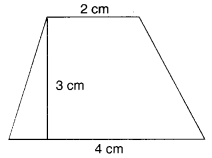(a) 9 cmē
(b) 6 cmē
(c) 7 cmē
(d) 24 cmē

Hint:
Area = $$\frac{(4+2)3}{2}$$ = 9 cmē.

Question 36.
The area of the trapezium is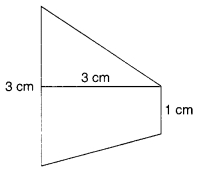(a) 6 cmē
(b) 4 cmē
(c) 3 cmē
(d) 9 cmē

Hint:
Area = $$\frac{(3+2)3}{2}$$ = 6 cmē.

Question 37.
The perimeter of the trapezium is(a) 12 cm
(b) 24 cm
(c) 6 cm
(d) 18 cm.

Hint:
Perimeter = 3 + 3 + 2 + 4 = 12 cm.

Question 38.
The area of the quadrilateral is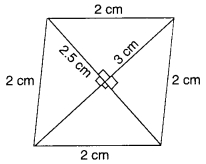(a) 3.75 cmē
(b) 7.5 cmē
(c) 3 cmē
(d) 10 cmē

Hint:
Area = $$\frac{1}{2}$$ Ũ 3 Ũ 2.5 = 1.5 Ũ 2.5
= 3.75 cmē.

Question 39.
The area of the quadrilateral is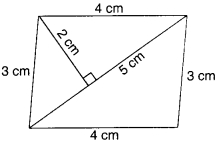(a) 10 cmē
(b) 5 cmē
(c) 20 cmē
(d) 15 cmē

Hint:
Area = 2($$\frac{5Ũ2}{2}$$) = 10 cmē.

Question 40.
The area of the quadrilateral is(a) 6 cmē
(b) 12 cmē
(c) 3 cmē
(d) 8 cmē

Hint:
Area = $$\frac{4Ũ(1+2)}{2}$$) = 6 cmē

Question 41.
The area of a rhombus is 60 cmē. One diagonal is 10 cm. The other diagonal is
(a) 6 cm
(b) 12 cm
(c) 3 cm
(d) 24 cm.

Hint:
$$\frac{1}{2}$$ Ũ 10 Ũ d2 = 60 ? d2 = 12 cm.

Question 42.
The area of a trapezium is 40 cmē. Its parallel sides are 12 cm and 8 cm. The distance between the parallel sides is
(a) 1 cm
(b) 2 cm
(c) 3 cm
(d) 4 cm.

Hint:
$$\frac{(12+8)d}{2}$$ = 40 ? d = 4 cm.

Question 43.
8 persons can stay in a cubical room. Each person requires 27 mģ of air. The side of the cube is
(a) 6 m
(b) 4 m
(c) 3 m
(d) 2 m.

Hint:
Volume = 8 Ũ 27 = 216 mģ
? side = $$\sqrt{216}$$ = 6 m.

Question 44.
If the height of a cuboid becomes zero, it will take the shape of a
(a) cube
(b) parallelogram
(c) circle
(d) rectangle.

Question 45.
The volume of a room is 80 mģ. The area of the floor is 20 mē. The height of the room is
(a) 1 m
(b) 2 m
(c) 3 m
(d) 4 m.

Hint:
Height = $$\frac{80}{20}$$ = 4 m.

Question 46.
The floor of a room is a square of side 6 m. Its height is 4 m. The volume of the room is
(a) 140 mģ
(b) 142 mģ
(c) 144 mģ
(d) 145 mģ

Hint:
Volume = 6 Ũ 6 Ũ 4 = 144 mģ.

Question 47.
The base radius and height of a right circular cylinder are 14 cm and 5 cm respectively. Its curved surface is
(a) 220 cmē
(b) 440 cmē
(c) 1232 cmē
(d) 2p Ũ 14 Ũ (14 + 5) cmē

Hint:
Curved surface = 2 Ũ $$\frac{22}{7}$$ Ũ 14 Ũ 5
= 440 cmē.

Question 48.
The heights of two right circular cylinders are the same. Their volumes are respectively 16p mģ and 81p mģ. The ratio of their base radii is
(a) 16 : 81
(b) 4 : 9
(c) 2 : 3
(d) 9 : 4.

Hint:
$$\frac{pr_1^2h}{pr_2^2h}$$ = $$\frac{16p}{81p}$$ ? $$\frac{r_1}{r_2}$$ = $$\frac{4}{9}$$

Question 49.
The ratio of the radii of two right circular cylinders is 1 : 2 and the ratio of their heights is 4 : 1. The ratio of their volumes is
(a) 1 : 1
(b) 1 : 2
(c) 2 : 1
(d) 4 : 1.

Hint:
$$\frac{r_1}{r_2}$$ = $$\frac{p(1)^24}{p(2)^21}$$ = 1 : 1

Question 50.
A glass in the form of a right circular cylinder is half full of water. Its base radius is 3 cm and height is 8 cm. The volume of water is
(a) 18p cmģ
(b) 36p cmģ
(c) 9p cmģ
(d) 36 cmģ

Hint:
Volume = $$\frac{1}{2}$$p Ũ 3 Ũ 3 Ũ 8 = 36p cmģ.

Question 51.
The base area of a right circular cylinder is 16K cmģ. Its height is 5 cm. Its curved surface area is
(a) 40p cmē
(b) 30p cmē
(c) 20p cmē
(d) 10p cmē

Hint:
prē = 16p ? r = 4 cm
? Curved surface area
= 2 Ũ p Ũ 4 Ũ 5 = 40p cmē

Question 52.
The base radius and height of a right circular cylinder are 5 cm and 10 cm. Its total surface area is
(a) 150p cmē
(b) 300p cmē
(c) 150 cmē
(d) 300 cmē

Hint:
Total surface area = 2pr (h + r)
= 2p 5 (10 + 5) = 150p cmē.

Question 53.
If the length and breadth of a rectangle are 10 cm and 5 cm, respectively, then its area is:
(a) 100 sq.cm
(b) 150 sq.cm
(c) 115 sq.cm
(d) 200 sq.cm

Hint:
Length = 10 cm
Area of rectangle = Lenght x breadth
= 10 x 5
= 150 cm2

Question 54.
The area of a rhombus whose diagonals are of lengths 10 cm and 8.2 cm is:
(a) 41 cm2
(b) 82 cm2
(c) 410 cm2
(d) 820 cm2

Hint:
Area of rhombus = ― d1 d2
A = ― x 10 x 8.2
A = 41 cm2

Question 55.
The area of a trapezium is 480 cm2, the distance between two parallel sides is 15 cm and one of the parallel side is 20 cm. The other parallel side is:
(a) 20 cm
(b) 34 cm
(c) 44 cm
(d) 50 cm

Hint:
Area of trapezium = ― h (a + b)
a=20 cm, h = 15 cm, Area = 480 sq.cm
480 = ― (15) (20 + b)
20 + b = (480 x 2)/15
20 + b = 64
b = 44cm

Question 56.
The area of a rhombus is 240 cm2 and one of the diagonals is 16 cm. Find the other diagonal.
(a) 16 cm
(b) 20 cm
(c) 30 cm
(d) 36 cm

Hint:
Area = 240 cm2
d1 = 16 cm
Area of rhombus = ― d1 x d2
240 = ― x 16 x d2
d2 = 480/16 = 30 cm

Question 57.
A cuboid has ______ pairs of identical faces.
(a) 2
(b) 3
(c) 4
(d) 5

Hint:
All six faces are rectangular, and opposites faces are identical. So there are three pairs of identical faces.

Question 58.
The area of a rectangle of length a and breadth b is
(a) a + b
(b) ab
(c) aē + bē
(d) 2ab.

Question 59.
The area of a square of side a is
(a)a
(b) aē
(c) 2a
(d) 4a.

Question 60.
The area of a triangle with base b and altitude h is
(a) $$\frac{1}{2}$$bh
(b) bh
(c) $$\frac{1}{3}$$bh
(d) $$\frac{1}{4}$$bh.

Answer: (a) $$\frac{1}{2}$$bh

Share: